Next: Updating the Experts Up: CEM - A Maximum Previous: Continuous Hidden Variables CEM

# CEM for Gaussian Mixture Models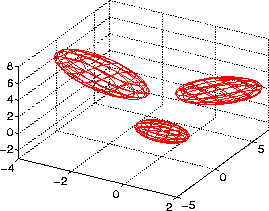Consider the Gaussian distribution in the mixture model in Figure 7.2. For the joint density case, Equation 7.13 depicts the mixture model. Here we are using the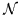definition to represent a multivariate normal (i.e. Gaussian) distribution. We can also consider an unnormalized Gaussian distribution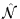shown in Equation 7.14. Equation 7.15 depicts the conditioned mixture model which is of particular interest for our estimation . In Equation 7.15 we also write the conditioned mixture of Gaussians in an experts and gates notation and utilize unnormalized Gaussian gates.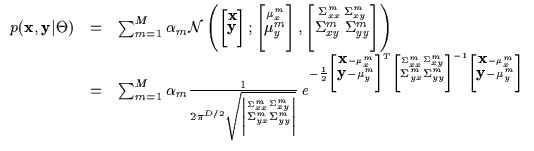(7.13)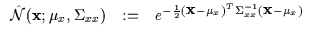(7.14)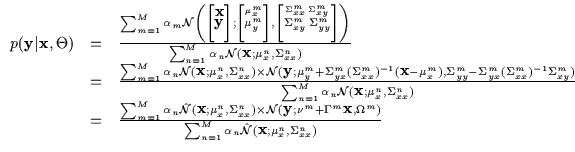(7.15)

The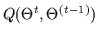function thus evolves into Equation 7.16. Note the use of a different parametrization for the experts: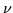is the conditional mean,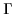is a regressor matrix and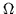is the conditional covariance. We immediately note that the experts and gates can be separated and treated independently since they are parametrized by independent variables (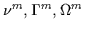versus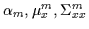). Both the gates and the experts can be varied freely and have no variables in common. In fact, we shall optimize these independently to maximize conditional likelihood. An iteration is performed over the experts and then an iteration over the gates. If each of those manipulations causes an increase, we will converge to a local maximum of conditional log-likelihood. This is similar in spirit to the ECM (Expectation Conditional Maximization) algorithm proposed in  since some variables are held constant while others are maximized and then vice-versa.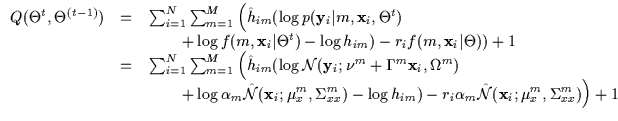(7.16)Next: Updating the Experts Up: CEM - A Maximum Previous: Continuous Hidden Variables CEM
Tony Jebara
1999-09-15